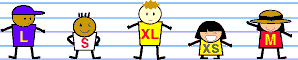# Mean Median Mode

Mean, median, and mode are introduced in this unit. Range and non-routine mean problems are also covered. Examples include problems with whole numbers, decimals and integers. Try our sample lessons below, or browse other units of instruction.Topics Description Range To find the range of a set of data. Whole numbers, integers, and decimals are used in the problems presented. All problems are drawn from real-life situations. Arithmetic Mean To define and compute the arithmetic mean. Whole numbers and decimals are used throughout. Rounding to a particular decimal place is required. Non-Routine Mean Students learn to work backwards by solving non-routine mean problems. For example, finding the missing number given the mean and the other numbers in the set. Whole numbers and decimals are used throughout. Median To find the median of a set of data. Sets with an odd and with an even number of items are explored. Whole numbers and decimals are used throughout. Mode To find the mode of a set of data. Bimodal sets are also covered. A distinction is made between a set with no mode and one with a mode of zero. Whole numbers, signed numbers, and decimals are used in the problems. Practice Exercises To complete 10 additional exercises as practice. To assess students' understanding of all concepts. Challenge Exercises To solve 10 additional problems that challenge students' understanding of all topics covered. Problems are drawn from real-life situations. To hone students' problem-solving skills. Solutions To review complete solutions to all exercises presented in this unit. Includes the problem, step-by-step solutions, final answer and units for each exercise. Learning Objectives Learn About Statistics Including Range, Mean, Median And Mode. Data Sets That Are Bimodal, Have No Mode, And With An Even Or An Odd Number Of Data.